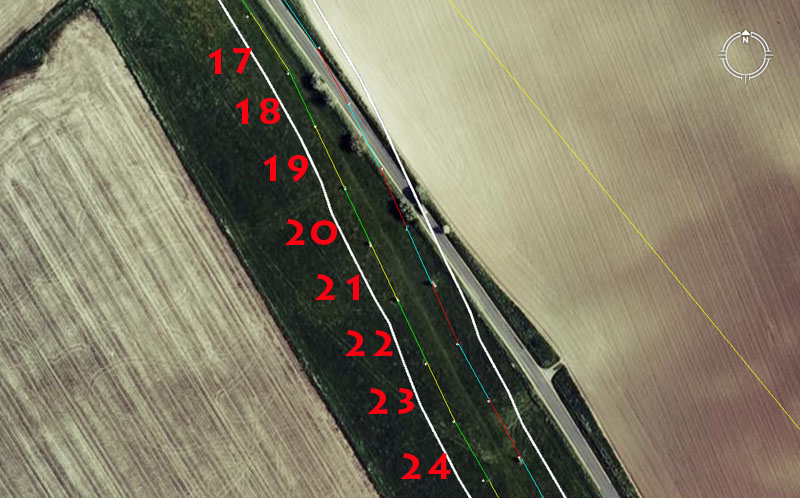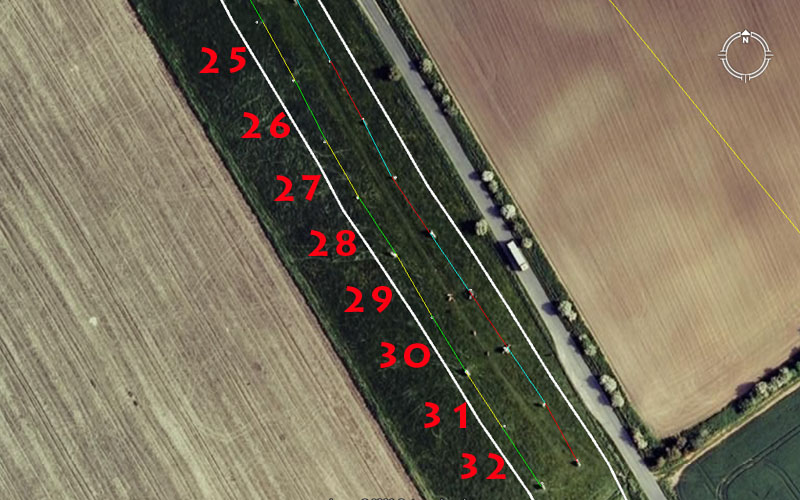SAILING FURTHER DOWN THE AVENUELeg 17.

The distance is 82.5-feet and the angle remains 146.66666-degrees.

The length is a repeat of leg 5 (5 rods or 15 ancient fathoms of 5.5-feet each). When converted to a circle, this length achieves a circumference of 259.2-feet or .72 of a foot per degree of arc or 18/25ths of a foot.

Leg 18.

The distance is 73.33333-feet and the angle is 154.6875-degrees.

For a world where the equatorial circumference was mathematically represented to be 24750-miles, the sum of 154.6875-miles (154 & 11/16ths) would be 1/160th of the circumference. This "11" series value used in the degree angle, when converted to a circumference using PI @ 3.141818182 = 486.This would mean that a 360-degree circle was broken down into 1.35 parts per degree of arc. Both the values 486 & 135 (lunar and navigational coding) formed very important mathematical progressions within the ancient system.

Leg 19.

The distance is 82.5-feet and the angle remains 154.6875.

Leg 20.

The distance reverts to 73.33333-feet and the angle remains 154.6875-degrees (154 & 11/16ths).

Leg 21.

The distance is 77-feet and the angle remains 154.6875-degrees.

Leg 22.

Again the distance reverts to 82.5-feet and the angle remains at 154.6875-degrees

Leg 23.

The distance is 79.2-feet (120 links of 7.92-inches each) and the angle remains 154.6875-degrees.

Leg 24.

The distance is 82.5-feet and the angle kinks eastwards to 150-degrees (or 330-degrees for the 180-degrees opposed angle back towards the henge).

In this section of eight legs there has been repetitive use of the same angle of heading. At the end of each leg the students would have, undoubtedly, read the changing angle to the crown position of Silbury Hill and noted the amount of variation. From such an exercise, over a long expanse, logarithmic charts of values could be made from which trigonometric calculations were achievable. The values derived could be recorded by coded knots on a string-knot device.Leg 25.

The distance is 77-feet and the angle is 151.25-degrees.

Under the Great Pyramid's second geodetic assignment, its length per side was symbolically represented to be 756.25-feet or 1512.5 for two side lengths. The value of 1512.5-feet represented 1/4th of 1-minute of equatorial arc under this "11" series reading. This angle value at West Kennet Avenue, if used as a diameter, would convert to a circumference of 475.2. In such a circle, each degree of arc would be 1.32 increases. The sum of 132-feet would be 1/40th of a mile.

Leg 26.

The distance is 77-feet and the angle shifts slightly westwards to 152.777777-degrees (152 & 7/9ths).

Using PI @ 3.141818182 this angle value converts to 480. In such a circle (480-feet for example) each degree of arc would be in 1.333333 increases. Again this is another vivid example of an "11" series value converting to something that could be adequately described as sexagesimal.

Leg 27.

The distance is 77-feet and the angle reverts slightly eastwards to 150-degrees (330-degrees opposed).

Leg 28.

The distance is 79.2-feet and the heading angle changes to 146.666666-degrees.

Leg 29.

The distance is 82.5-feet and the angle reverts westwards to 150-degrees (330-degrees opposed).

Leg 30.

The distance is 77-feet and the angle is 148.5-degrees.

The angle now swings westwards slightly. The sum of 148.5, if read as miles would be 1/166.666666ths of the 24750-mile equatorial circumference. It will be remembered that the 583.333333-feet distance between the (formerly huge) central obelisk position in the henge and the outlying obelisk standing at the southern gate at the entrance to the West Kennet Avenue is set at an azimuth angle of 166.6666666-degrees. In any 3.4.5 triangle the adjacent length is 1.6666666 less in length than the hypotenuse.

The sum of 148.5, if used as a diameter and converted to a circumference using PI @ 3.141818182 would achieve 466.56. It will be remembered that a marker sits on the Stonehenge Avenue that expresses 233.28-feet of distance from the centre of Stonehenge for a circle diameter of 466.56-feet. In such a circle 1/360th part would be 1.296-feet. The outer face of the Heel Stone at Stonehenge sits 129.6-feet from site centre and a mathematical progression based upon 1296 was very important to many ancient calculations (Precession of the Equinoxes and equatorial circumference of the Earth under the 24883.2-mile assignment, etc.).

Leg 31.

The distance is 77-feet and the angle is 146.666666-degrees.

Leg 32.

The distance is 82.5-feet and the angle remains 146.66666-degrees.

We have now done 32 legs of this line on West Kennet Avenue, but aren't yet even half way to the Sanctuary Circle. We could carry on for a few more positions until the occurrence of stone markers becomes erratic or peters out for long runs then spasmodically recur. Enough positions have been analysed to show what's going on down this particular line and we can reconsider what's left at a later date.

It's very important for the reader to realise that the AutoCAD lines seen to be going from stone to stone are linked together and continuous. This has not been a case of leaving little gaps or fudging angles to make things work. There can be no doubt that the foregoing represents the true design intention of the original architects.

We'll now return to the henge and start all over again, except next time we'll come down the other line further east and do all of our navigational calculations using the "6&7" system (based upon a mile of 5250-feet).

Continue